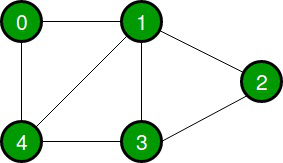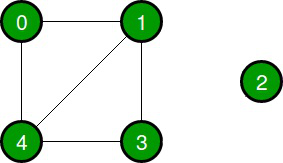Open in App
Not now

# Java Program to Check Whether Undirected Graph is Connected Using DFS

• Difficulty Level : Medium
• Last Updated : 02 Feb, 2021

Given an undirected graph, the task is to check if the given graph is connected or not using DFS.

A connected graph is a graph that is connected in the sense of a topological space, i.e., there is always a path from any node to any other node in the graph. A graph that is not connected is said to be disconnected.

Examples:

Input:Output: Graph is connected

Input:Output: Graph is disconnected

Approach:

1. Take a boolean visited [] array.
2. Start DFS(Depth First Search) from any of the vertexes and mark the visited vertices as True in the visited[] array.
3. After completion of DFS check if all the vertices in the visited [] array is marked as True.
4. If yes then the graph is connected, or else the graph is not connected or disconnected.

Code:

## Java

 `// Java Program to check if ``// an undirected graph is connected or not``// using DFS`` ` `import` `java.util.*; `` ` `public` `class` `checkConnectivity {``     ` `    ``// Graph class``    ``static` `class` `Graph{``         ` `        ``int` `vertices;``        ``// Linked list for adjacency list of a vertex``        ``LinkedList adjacencyList [];`` ` `        ``@SuppressWarnings``(``"unchecked"``)``        ``public` `Graph(``int` `vertices)``        ``{``            ``this``.vertices = vertices;``            ``adjacencyList = ``new` `LinkedList[vertices];``           ` `            ``for` `(``int` `i = ``0``; i();``            ``}``        ``}``         ` `        ``// Function for adding edges``        ``public` `void` `addEdge(``int` `source, ``int` `dest)``        ``{``            ``adjacencyList.addFirst(dest);``            ``adjacencyList[dest].addFirst(source);``        ``}``    ``}`` ` `    ``// Function to check if the graph is connected or not``    ``public` `void` `isConnected(Graph graph){`` ` `        ``int` `vertices = graph.vertices;``        ``LinkedList adjacencyList [] = graph.adjacencyList;`` ` `        ``// Take a boolean visited array``        ``boolean``[] visited = ``new` `boolean``[vertices];`` ` `        ``// Start the DFS from vertex 0``        ``DFS(``0``, adjacencyList, visited);`` ` `        ``// Check if all the vertices are visited``        ``// Set connected to False if one node is unvisited``        ``boolean` `connected = ``true``;``       ` `        ``for` `(``int` `i = ``0``; i adjacencyList [], ``boolean``[] visited){`` ` `        ``// Mark the vertex visited as True``        ``visited = ``true``;`` ` `        ``// Travel the adjacent neighbours``        ``for` `(``int` `i = ``0``; i

Output

```Graph 1:- Graph is connected
Graph 2:- Graph is disconnected
```

My Personal Notes arrow_drop_up